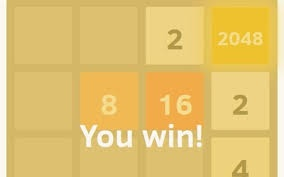# Science of Apps! - Part 3: 2048

Probability Level 4$2048$ is an insanely addictive game. The objective is simple, bring the same powers of two together to add them together, and keep increasing the powers of $2$ until you reach $2048$ and beyond.

The game starts with $2$ and $4$ tiles, popping up in different places of the $4\times 4$ grid.

To get to the $2048$ tile requires finesse, and a slight bit of luck. In the best scenario, what is the least number of moves required to get the $2048$ tile?

This problem is part of the Science of Apps! series

×